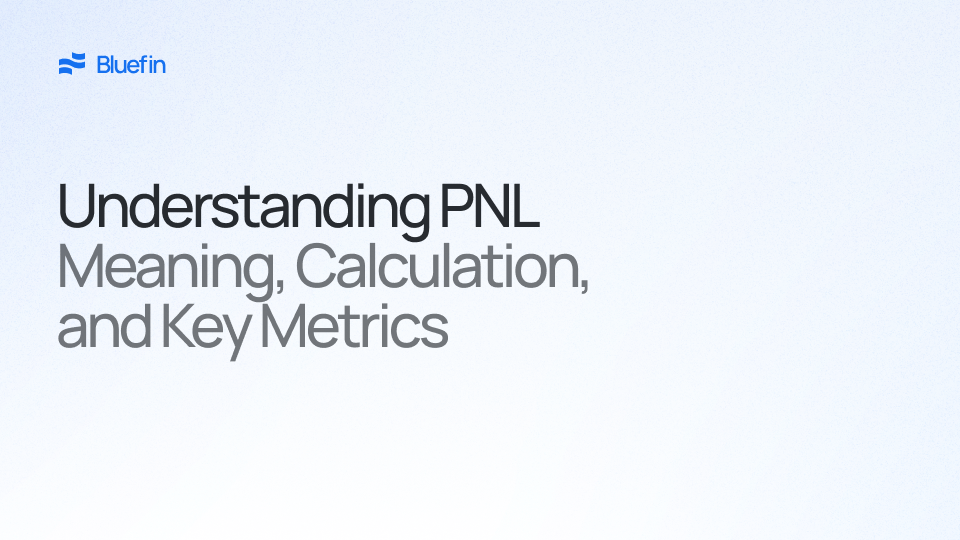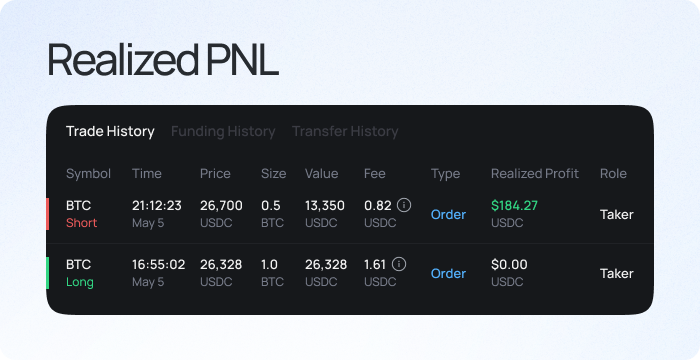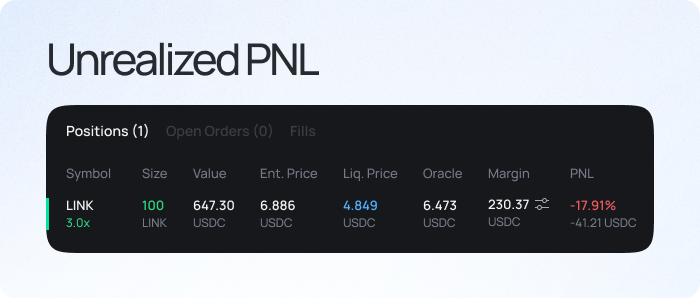## What is PnL?

Profit and Loss (PnL or P&L) is a critical financial metric used to determine the net profit or loss of an individual or company over a specific period. It shows how much net profit an entity has generated over a time period or in a certain number of transactions. Understanding PnL is essential for investors, traders, and business owners.

## Realized PnL

Realized PnL is the profit or loss generated from a completed or closed trade. It is essential for evaluating the effectiveness of a trading strategy and required in tax calculations, as realized profits are subject to capital gains tax, while realized losses can offset capital gains and reduce the tax liability.Realized PnL Displayed on Bluefin Exchange Account History Page

### Calculation

Without taking commissions or gas fees into account for simplicity, realized PnL is calculated as:

```Long Trade = Size Closed × (Exit Price − Entry Price)Short Trade = Size Closed × (Entry Price − Exit Price)```

A trader buys/longs an asset at a price of \$10 and closes the trade at \$15. The realized PnL would be \$5 (or \$5 realized profit).

`1 x (\$15 - \$10) = \$5`

However, if the price dropped to \$7 & the trader closed the trade, the PnL would be -\$3 (or \$3 realized loss).

`1 x (\$7 - \$10) = -\$3`

In contrast, if a user sells/shorts an asset at a price of \$10 and closes the trade at \$15. The realized PnL would be -\$5 (or \$5 realized loss).

`1 x (\$10 - \$15) = -\$5`

However, if the price dropped to \$7 & the trader closed the trade, the PnL would be \$3 (or \$3 realized profit).

`1 x (\$10 - \$7) = \$3`

## Unrealized PnL

Unrealized PnL is the profit or loss generated from an open position or trade that has yet to be closed. It represents the difference between an asset's current market value (Index price) and purchase (entry) price. Unrealized PnL is also known as "paper profit or loss" because the position has not yet closed. If the trader decides to close the trade at the current market price, the unrealized PnL becomes realized PnL.Unrealized PnL Displayed on Bluefin Exchange Open Positions

### Calculation

Without taking commissions or gas fees into account for simplicity, unrealized PnL is calculated as:

```Long Trade = Position Size × (Index Price − Entry Price)Short Trade = Position Size × (Entry Price − Index Price)```

## What is PnL Percentage?

PnL Percentage refers to the profit and loss relative to the initial money ‘at-risk’, which is another way to measure the relative gain or loss on an investment or trading position. PnL percentage is most used to analyze and compare the performance of trading strategies.

To calculate the PnL Percentage, you need:

• The initial investment value, or Starting Value and
• the Final Value of the investment.

The formula to calculate PnL Percentage is as follows:

`PnL Percentage = (Final Value/ Starting Value - 1) * 100%`

The formula yields PnL relative compared to your initial investment. A positive percentage means a profit was made, while a negative percentage indicates a loss.

### Example

A trader buys/longs an asset at an entry price of \$10 and closes that trade when the price of the asset is \$15. The PnL percentage will be 50%.

`(\$15/\$10 - 1) * 100% = 50%`

## What is PnL Ratio

The PnL Ratio is also a measure of a trader’s performance that takes into account the size of their profits and losses relative to their trading capital. It is calculated by dividing the total profits by the total losses.

The formula to calculate PnL Ratio is as follows:

`PnL Ratio = Total Profits / Total Losses`

A PnL Ratio greater than 1 indicates that the trader has made more profit than loss, while a PnL Ratio less than 1 indicates that the trader has made more loss than profit.

### Example

If a trader has made \$10,000 in profits and \$5,000 in losses, the PnL Ratio would be:

`PnL Ratio = \$10,000 / \$5,000PnL Ratio = 2`

This indicates that the trader has made twice as much profit as loss, which is a good sign.

In conclusion, PnL is an essential metric for traders, investors, and business owners to evaluate their trading strategy, financial performance, and tax liability. By understanding the different ways of calculating and evaluating PnL, such as PnL Percentage, and PnL Ratio, individuals and companies can make informed decisions about their financial strategies and performance.